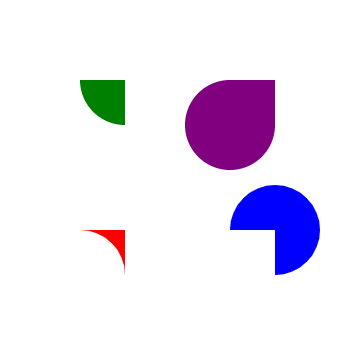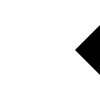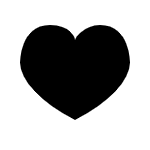# Drawing paths#

There are two ways for creating and drawing a path in ipycanvas.

## Using Path2D#

You can define a Path2D given an SVG path. Note that once the path is created, it is read only, you cannot dynamically change the path value. Using the Path2D class is very useful and efficient when you want to reuse the same path multiple times.

See https://developer.mozilla.org/en-US/docs/Web/SVG/Tutorial/Paths for documentation about SVG paths.

• `Path2D(value)`:

Creates a Path2D given the SVG path string value.

```from ipycanvas import Canvas, Path2D

canvas = Canvas(width=350, height=350)

path1 = Path2D("M80 80 A 45 45, 0, 0, 0, 125 125 L 125 80 Z")
path2 = Path2D("M230 80 A 45 45, 0, 1, 0, 275 125 L 275 80 Z")
path3 = Path2D("M80 230 A 45 45, 0, 0, 1, 125 275 L 125 230 Z")
path4 = Path2D("M230 230 A 45 45, 0, 1, 1, 275 275 L 275 230 Z")

canvas.fill_style = "green"
canvas.fill(path1)

canvas.fill_style = "purple"
canvas.fill(path2)

canvas.fill_style = "red"
canvas.fill(path3)

canvas.fill_style = "blue"
canvas.fill(path4)

canvas
```## Using Path commands#

A path is a list of points, connected by segments of lines that can be of different shapes, curved or not, of different width and of different color. A path can be closed. To make shapes using paths, we take some extra steps:

• First, you create the path with `begin_path`

• Then you use drawing commands to add shapes into the path

• Once the path has been created, you can `stroke` or `fill` the path to render it

Here are the functions used to perform these steps:

• `begin_path()`:

Creates a new path. Once created, future drawing commands are directed into the path and used to build the path up.

• Path commands like `line_to` and `arc`

• `close_path()`:

Adds a straight line to the path, going to the start of the current path.

• `stroke()`:

Draws the shape by stroking its outline.

• `fill(rule)`:

Draws a solid shape by filling the path’s content area. The given fill rule is applied, possible rules are nonzero and evenodd.

```from ipycanvas import Canvas

canvas = Canvas(width=100, height=100)

# Draw simple triangle shape
canvas.begin_path()
canvas.move_to(75, 50)
canvas.line_to(100, 75)
canvas.line_to(100, 25)
canvas.fill()

canvas
```### Path commands#

Here are the available draw commands:

• `move_to(x, y)`:

Moves the pen to the coordinates specified by x and y. This does not actually draw anything.

• `line_to(x, y)`:

Add a straight line to the current path by connecting the path’s last point to the specified (x, y) coordinates.

• `arc(x, y, radius, start_angle, end_angle, anticlockwise=False)`:

Add a circular arc centered at (x, y) with a radius of `radius` to the current path. The path starts at `start_angle` and ends at `end_angle` in radians, and travels in the direction given by `anticlockwise` (defaulting to clockwise: False).

• `arc_to(x1, y1, x2, y2, radius)`:

Add a circular arc to the current path. Using the given control points (`x1`, `y1`) and (`x2`, `y2`) and the `radius`.

• `ellipse(x, y, radius_x, radius_y, rotation, start_angle, end_angle, anticlockwise=False)`:

Add an ellipse centered at `(x, y)` with the radii `radius_x` and `radius_y` to the current path.

• `quadratic_curve_to(cp1x, cp1y, x, y)`:

Add a quadratic Bezier curve to the current path. It requires two points: the first one is a control point and the second one is the end point. The starting point is the latest point in the current path, which can be changed using `move_to()` before creating the quadratic Bezier curve.

• `bezier_curve_to(cp1x, cp1y, cp2x, cp2y, x, y)`:

Add a cubic Bezier curve to the current path. It requires three points: the first two are control points and the third one is the end point. The starting point is the latest point in the current path, which can be changed using `move_to()` before creating the Bezier curve.

• `rect(x, y, width, height)`:

Draws a rectangle whose top-left corner is specified by (`x`, `y`) with the specified `width` and `height`.

### Examples#

#### Stroke arcs#

```from math import pi

from ipycanvas import Canvas

canvas = Canvas(width=200, height=200)

# Draw smiley face
canvas.begin_path()
canvas.arc(75, 75, 50, 0, pi * 2, True)  # Outer circle
canvas.move_to(110, 75)
canvas.arc(75, 75, 35, 0, pi, False)  # Mouth (clockwise)
canvas.move_to(65, 65)
canvas.arc(60, 65, 5, 0, pi * 2, True)  # Left eye
canvas.move_to(95, 65)
canvas.arc(90, 65, 5, 0, pi * 2, True)  # Right eye
canvas.stroke()

canvas
```#### Fill bezier curves#

```from ipycanvas import Canvas

canvas = Canvas(width=200, height=200)

# Cubic curves example
canvas.begin_path()
canvas.move_to(75, 40)
canvas.bezier_curve_to(75, 37, 70, 25, 50, 25)
canvas.bezier_curve_to(20, 25, 20, 62.5, 20, 62.5)
canvas.bezier_curve_to(20, 80, 40, 102, 75, 120)
canvas.bezier_curve_to(110, 102, 130, 80, 130, 62.5)
canvas.bezier_curve_to(130, 62.5, 130, 25, 100, 25)
canvas.bezier_curve_to(85, 25, 75, 37, 75, 40)
canvas.fill()

canvas
```#### Change the fill rule#

```from math import pi
from ipycanvas import Canvas

canvas = Canvas(width=100, height=100)

canvas.begin_path()
canvas.arc(50, 50, 30, 0, pi * 2, True)
canvas.arc(50, 50, 15, 0, pi * 2, True)
canvas.fill("evenodd")

canvas
```